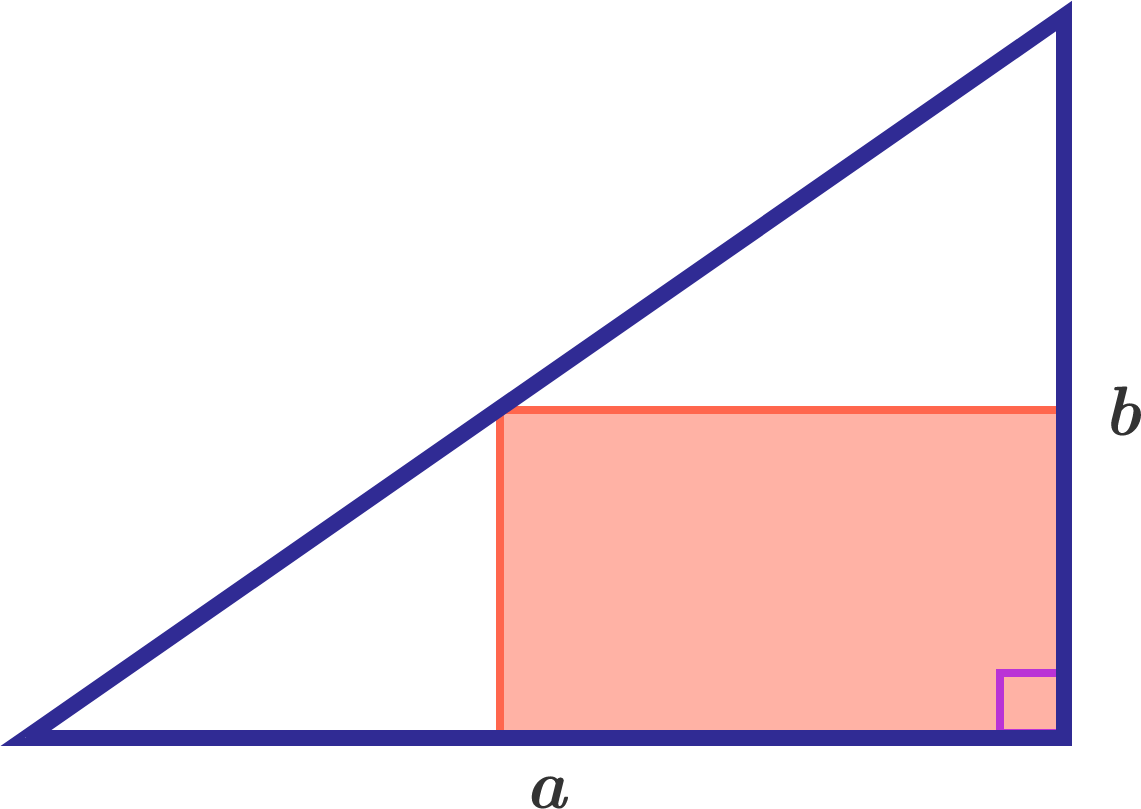# Rectangle in triangle

Calculus Level 2

A rectangle is drawn inside a right-angled triangle so that two of its sides lie on the shorter sides of the triangle. If the shorter sides of the triangle have lengths $a$ and $b,$ what is the maximum area of the rectangle in terms of $a$ and $b?$×

Problem Loading...

Note Loading...

Set Loading...